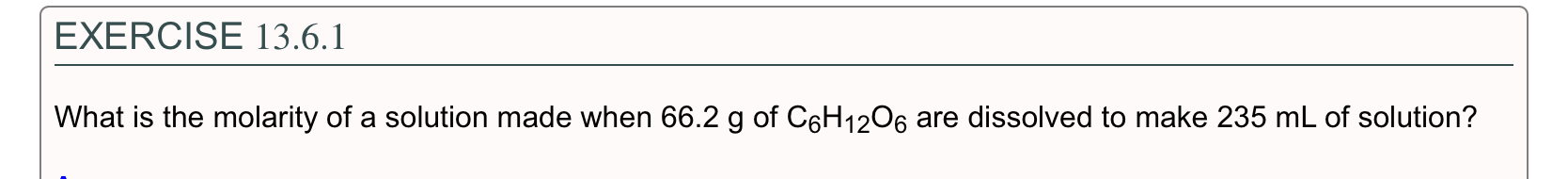# EXERCISE 13.6.1What is the molarity of a solution made when 66.2 g of C6H12O6 are dissolved to make 235 mL of solution?

Questionhelp_outlineImage TranscriptioncloseEXERCISE 13.6.1 What is the molarity of a solution made when 66.2 g of C6H12O6 are dissolved to make 235 mL of solution? fullscreen
check_circle

Step 1

The molarity of a solution is the ratio of number of moles of a substance to the volume of solution in liters. The expression for molarity is labelled in equation (1) in which M is the molarity, n is the number of moles of solute and  V is the volume in liters.

The number of moles of a substance is the ratio of its given mass by molar mass of the substance. It is expressed in equation (2) in which n is the number of moles, m is the given mass and M is the molar mass.

The relation between millilitres (mL) and liters (L) is written below:

1 mL= 0.001 L    …… (3)

Step 2

The molar mass of C6H12O6 is 180.156 g/mol. Use equation (2) to determine the number of moles of C6H12O6.

Step 3

Use equation (3) to convert 235 mL into liters.

235 mL = 0.235 L

The molar...

### Want to see the full answer?

See Solution

#### Want to see this answer and more?

Solutions are written by subject experts who are available 24/7. Questions are typically answered within 1 hour.*

See Solution
*Response times may vary by subject and question.
Tagged in

### General Chemistry